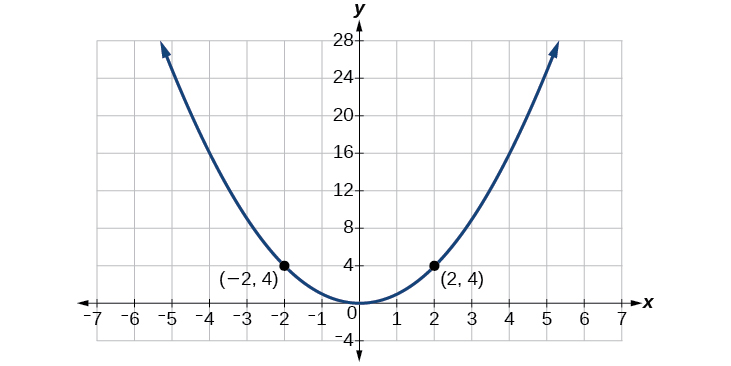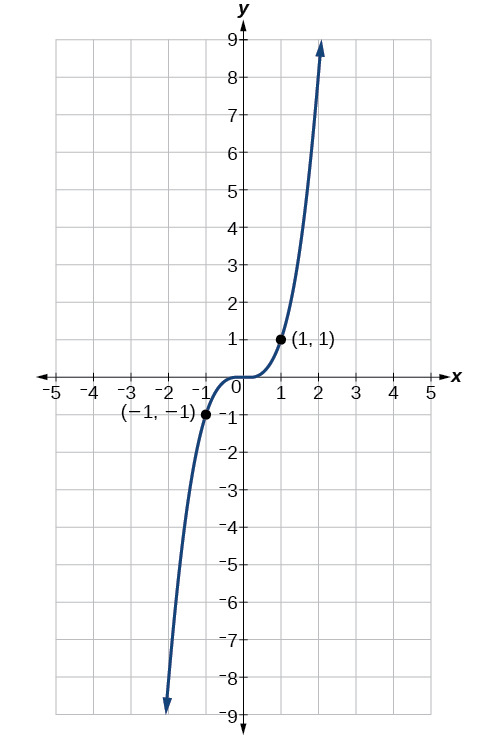# 7.4 The other trigonometric functions  (Page 3/14)

 Page 3 / 14

Use reference angles to find all six trigonometric functions of $\text{\hspace{0.17em}}-\frac{7\pi }{4}.$

$\begin{array}{l}\\ \mathrm{sin}\left(\frac{-7\pi }{4}\right)=\frac{\sqrt{2}}{2},\mathrm{cos}\left(\frac{-7\pi }{4}\right)=\frac{\sqrt{2}}{2},\mathrm{tan}\left(\frac{-7\pi }{4}\right)=1,\\ \mathrm{sec}\left(\frac{-7\pi }{4}\right)=\sqrt{2},\mathrm{csc}\left(\frac{-7\pi }{4}\right)=\sqrt{2},\mathrm{cot}\left(\frac{-7\pi }{4}\right)=1\end{array}$

## Using even and odd trigonometric functions

To be able to use our six trigonometric functions freely with both positive and negative angle inputs, we should examine how each function treats a negative input. As it turns out, there is an important difference among the functions in this regard.

Consider the function $\text{\hspace{0.17em}}f\left(x\right)={x}^{2},$ shown in [link] . The graph of the function is symmetrical about the y -axis. All along the curve, any two points with opposite x -values have the same function value. This matches the result of calculation: $\text{\hspace{0.17em}}{\left(4\right)}^{2}={\left(-4\right)}^{2},{\left(-5\right)}^{2}={\left(5\right)}^{2},$ and so on. So $\text{\hspace{0.17em}}f\left(x\right)={x}^{2}\text{\hspace{0.17em}}$ is an even function, a function such that two inputs that are opposites have the same output. That means $\text{\hspace{0.17em}}f\left(-x\right)=f\left(x\right).$The function   f ( x ) = x 2   is an even function.

Now consider the function $\text{\hspace{0.17em}}f\left(x\right)={x}^{3},$ shown in [link] . The graph is not symmetrical about the y -axis. All along the graph, any two points with opposite x -values also have opposite y -values. So $\text{\hspace{0.17em}}f\left(x\right)={x}^{3}\text{\hspace{0.17em}}$ is an odd function, one such that two inputs that are opposites have outputs that are also opposites. That means $\text{\hspace{0.17em}}f\left(-x\right)=-f\left(x\right).$The function   f ( x ) = x 3   is an odd function.

We can test whether a trigonometric function is even or odd by drawing a unit circle with a positive and a negative angle, as in [link] . The sine of the positive angle is $\text{\hspace{0.17em}}y.\text{\hspace{0.17em}}$ The sine of the negative angle is $\text{\hspace{0.17em}}-y.\text{\hspace{0.17em}}$ The sine function, then, is an odd function. We can test each of the six trigonometric functions in this fashion. The results are shown in [link] .

## Even and odd trigonometric functions

An even function is one in which $\text{\hspace{0.17em}}f\left(-x\right)=f\left(x\right).$

An odd function is one in which $\text{\hspace{0.17em}}f\left(-x\right)=-f\left(x\right).$

Cosine and secant are even:

Sine, tangent, cosecant, and cotangent are odd:

## Using even and odd properties of trigonometric functions

If the secant of angle $\text{\hspace{0.17em}}t\text{\hspace{0.17em}}$ is 2, what is the secant of $\text{\hspace{0.17em}}-t?$

Secant is an even function. The secant of an angle is the same as the secant of its opposite. So if the secant of angle $\text{\hspace{0.17em}}t\text{\hspace{0.17em}}$ is 2, the secant of $\text{\hspace{0.17em}}-t\text{\hspace{0.17em}}$ is also 2.

If the cotangent of angle $\text{\hspace{0.17em}}t\text{\hspace{0.17em}}$ is $\text{\hspace{0.17em}}\sqrt{3},$ what is the cotangent of $\text{\hspace{0.17em}}-t?$

$-\sqrt{3}$

## Recognizing and using fundamental identities

We have explored a number of properties of trigonometric functions. Now, we can take the relationships a step further, and derive some fundamental identities. Identities are statements that are true for all values of the input on which they are defined. Usually, identities can be derived from definitions and relationships we already know. For example, the Pythagorean Identity    we learned earlier was derived from the Pythagorean Theorem and the definitions of sine and cosine.

## Fundamental identities

We can derive some useful identities    from the six trigonometric functions. The other four trigonometric functions can be related back to the sine and cosine functions using these basic relationships:

$\mathrm{tan}\text{\hspace{0.17em}}t=\frac{\mathrm{sin}\text{\hspace{0.17em}}t}{\mathrm{cos}\text{\hspace{0.17em}}t}$
$\mathrm{sec}\text{\hspace{0.17em}}t=\frac{1}{\mathrm{cos}\text{\hspace{0.17em}}t}$
$\mathrm{csc}\text{\hspace{0.17em}}t=\frac{1}{\mathrm{sin}\text{\hspace{0.17em}}t}$
$\text{cot}\text{\hspace{0.17em}}t=\frac{1}{\text{tan}\text{\hspace{0.17em}}t}=\frac{\text{cos}\text{\hspace{0.17em}}t}{\text{sin}\text{\hspace{0.17em}}t}$

#### Questions & Answers

x exposant 4 + 4 x exposant 3 + 8 exposant 2 + 4 x + 1 = 0
x exposent4+4x exposent3+8x exposent2+4x+1=0
HERVE
How can I solve for a domain and a codomains in a given function?
ranges
EDWIN
Thank you I mean range sir.
Oliver
proof for set theory
don't you know?
Inkoom
find to nearest one decimal place of centimeter the length of an arc of circle of radius length 12.5cm and subtending of centeral angle 1.6rad
factoring polynomial
find general solution of the Tanx=-1/root3,secx=2/root3
find general solution of the following equation
Nani
the value of 2 sin square 60 Cos 60
0.75
Lynne
0.75
Inkoom
when can I use sin, cos tan in a giving question
depending on the question
Nicholas
I am a carpenter and I have to cut and assemble a conventional roof line for a new home. The dimensions are: width 30'6" length 40'6". I want a 6 and 12 pitch. The roof is a full hip construction. Give me the L,W and height of rafters for the hip, hip jacks also the length of common jacks.
John
I want to learn the calculations
where can I get indices
I need matrices
Nasasira
hi
Raihany
Hi
Solomon
need help
Raihany
maybe provide us videos
Nasasira
Raihany
Hello
Cromwell
a
Amie
What do you mean by a
Cromwell
nothing. I accidentally press it
Amie
you guys know any app with matrices?
Khay
Ok
Cromwell
Solve the x? x=18+(24-3)=72
x-39=72 x=111
Suraj
Solve the formula for the indicated variable P=b+4a+2c, for b
Need help with this question please
b=-4ac-2c+P
Denisse
b=p-4a-2c
Suddhen
b= p - 4a - 2c
Snr
p=2(2a+C)+b
Suraj
b=p-2(2a+c)
Tapiwa
P=4a+b+2C
COLEMAN
b=P-4a-2c
COLEMAN
like Deadra, show me the step by step order of operation to alive for b
John
A laser rangefinder is locked on a comet approaching Earth. The distance g(x), in kilometers, of the comet after x days, for x in the interval 0 to 30 days, is given by g(x)=250,000csc(π30x). Graph g(x) on the interval [0, 35]. Evaluate g(5)  and interpret the information. What is the minimum distance between the comet and Earth? When does this occur? To which constant in the equation does this correspond? Find and discuss the meaning of any vertical asymptotes.
The sequence is {1,-1,1-1.....} hasByByByByBy David Martin